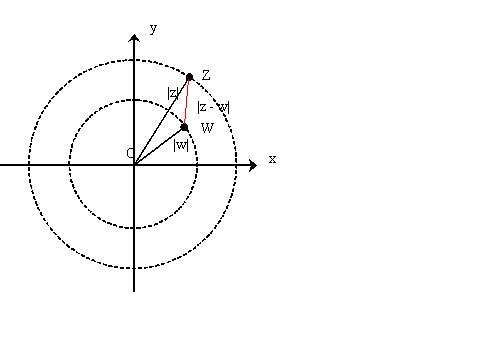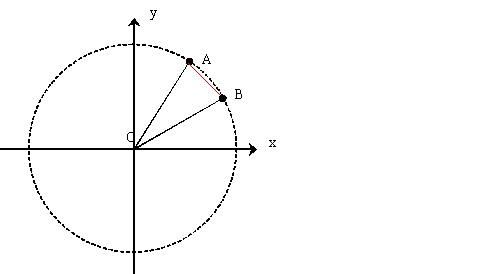#### You may also like### Roots and Coefficients

If xyz = 1 and x+y+z =1/x + 1/y + 1/z show that at least one of these numbers must be 1. Now for the complexity! When are the other numbers real and when are they complex?### Target Six

Show that x = 1 is a solution of the equation x^(3/2) - 8x^(-3/2) = 7 and find all other solutions.### 8 Methods for Three by One

This problem in geometry has been solved in no less than EIGHT ways by a pair of students. How would you solve it? How many of their solutions can you follow? How are they the same or different? Which do you like best?

# Thousand Words

##### Age 16 to 18Challenge Level

Thank you to Roy M. from Allerton High School and Andrei L. from Tudor Vianu National College, Bucharest, Romania for their solutions.

Draw two concentric circles with centres at the origin and radii $|z|$ and $|w|$ and suppose, without loss of generality, that $|z|> |w|$ then $z$ lies somewhere on the outer circle and $w$ lies somewhere on the inner circle.In this representation, working with complex numbers is similar to working with vectors. The two complex numbers can be added and subtracted as vectors. So, to subtract them, it is the same as adding one to the opposite of the other. The result is represented in the figure. The distance between the two points representing the complex numbers $z$ and $w$ is the modulus of $z-w$ written as $|z-w|$. The lengths of the sides of the triangle in the diagram are $|z|$, $|w|$ and $|z-w|$.

The shortest distance between any two points where one is on each circle is given by the difference of the radii of the two circles $|z|-|w|$. This would be the distance between the points $z$ and $w$ if they had the same argument. If $z$ and $w$ have different arguments then the distance between the points will be $|z- w|$ and hence $|z-w|\geq |z| - |w|$.

For the second part, mark points $A$ and $B$ on the unit circle to represent $e^{i\alpha*}$ and $e^{i\beta*}$ respectively where $\alpha*$ and $\beta*$ lie between 0 and $2\pi$. To include all possible values of $\alpha$ and $\beta$ we have $\alpha =\alpha* +2m\pi$ and $\beta = \beta* + 2n\pi$ for some integers $m$ and $n$. Clearly $A$ and $B$ also represent $e^{i\alpha}$ and $e^{i\beta}.$The chord length $AB$ is less than or equal to the arc length $AB$. Hence $$|e^{i\alpha}-e^{i\beta}|\leq \alpha - \beta.$$